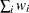PROC STDIZE Statement

PROC STDIZE <options> ;

The PROC STDIZE statement invokes the procedure. You can specify the following options in the PROC STDIZE statement. Table 84.1 summarizes the options.

Table 84.1 Summary of PROC STDIZE Statement Options

Option

Description

Specify standardization methods

Specifies the name of the standardization method

Specifies the method for computing initial estimates for the A estimates

Unstandardize variables

Unstandardizes variables when you also specify the METHOD=IN option

Process missing values

Omits observations with any missing values from computation

Specifies the method or a numeric value for replacing missing values

Replaces missing data with zero in the standardized data

Replaces missing data with the location measure (does not standardize the data)

Specify data set details

Specifies the input data set

Specifies that output variables inherit the length of the analysis variable

Specifies the output data set

Specifies that original variables appear in the OUT= data set

Specifies a prefix for the standardized variable names

Specifies the output statistic data set

Specify computational settings

Specifies the variances divisor

Specifies the number of markers when you also specify PCTLMTD=ONEPASS

Specifies the constant to multiply each value by after standardizing

Specifies the constant to add to each value after standardizing and multiplying by the value specified in the MULT= option

Specifies the relative fuzz factor for writing the output

Specify percentiles

Specifies the definition of percentiles when you also specify the PCTLMTD=ORD_STAT option

Specifies the method used to estimate percentiles

Writes observations containing percentiles to the data set specified in the OUTSTAT= option

Normalize scale estimators

Normalizes the scale estimator to be consistent for the standard deviation of a normal distribution

Normalizes the scale estimator to have an expectation of approximately 1 for a standard normal distribution

Specify output

Displays the location and scale measures

These options and their abbreviations are described (in alphabetical order) in the remainder of this section.

specifies a constant, c, to add to each value after standardizing and multiplying by the value you specify in the MULT= option. The default value is 0.

DATA=SAS-data-set

specifies the input data set to be standardized. If you omit the DATA= option, the most recently created data set is used.

FUZZ=c

specifies the relative fuzz factor. The default value is 1E–14. For the OUT= data set, the score is computed as follows: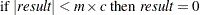where m is the constant specified in the MULT= option, or 1 if MULT= option is not specified.

For the OUTSTAT= data set and the location and scale table, the scale and location values are computed as follows: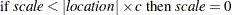Otherwise,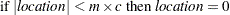INITIAL=method

specifies the method for computing initial estimates for the A estimates (ABW, AWAVE, and AHUBER). You cannot specify the following methods for initial estimates: INITIAL=ABW, INITIAL=AHUBER, INITIAL=AWAVE, and INITIAL=IN. The default is INITIAL=MAD.

KEEPLEN

specifies that the standardized variables inherit the lengths of the analysis variables that PROC STDIZE uses to derive them. PROC STDIZE stores numbers in double-precision without this option.

Caution: The KEEPLEN option causes the standardized variables to permanently lose numeric precision by truncating or rounding the values. However, the precision of the output variables will match that of the input.

METHOD=name

specifies the name of the method for computing location and scale measures. Valid values for name are as follows: MEAN, MEDIAN, SUM, EUCLEN, USTD, STD, RANGE, MIDRANGE, MAXABS, IQR, MAD, ABW, AHUBER, AWAVE, AGK, SPACING, L, and IN.

For details about these methods, see the descriptions in the section Standardization Methods. The default is METHOD=STD.

MISSING=method | value

specifies the method (or a numeric value) for replacing missing values. If you omit the MISSING= option, the REPLACE option replaces missing values with the location measure given by the METHOD= option. Specify the MISSING= option when you want to replace missing values with a different value. You can specify any name that is valid in the METHOD= option except the name IN. The corresponding location measure is used to replace missing values.

If a numeric value is given, the value replaces missing values after standardizing the data. However, you can specify the REPONLY option with the MISSING= option to suppress standardization for cases in which you want only to replace missing values.

MULT=c

specifies a constant, c, by which to multiply each value after standardizing. The default value is 1.

NMARKERS=n

specifies the number of markers used when you specify the one-pass algorithm (PCTLMTD=ONEPASS). The value n must be greater than or equal to 5. The default value is 105.

NOMISS

omits observations with missing values for any of the analyzed variables from calculation of the location and scale measures. If you omit the NOMISS option, all nonmissing values are used.

NORM

normalizes the scale estimator to be consistent for the standard deviation of a normal distribution when you specify the option METHOD=AGK, METHOD=IQR, METHOD=MAD, or METHOD=SPACING.

OPREFIX<=o-prefix>

specifies that the original variables should appear in the OUT= data set. You can optionally specify an equal sign and a prefix. For example, if OPREFIX=Original, then the names of the variables are OriginalVAR1, OriginalVAR2, and so on, where VAR1 and VAR2 are the original variable names. The value of OPREFIX= must be different from the value of SPREFIX=. If you specify OPREFIX, without an equal sign and a prefix, then the default prefix is null and you must specify SPREFIX=s-prefix.

OUT=SAS-data-set

specifies the name of the SAS data set created by PROC STDIZE. By default, the output data set is a copy of the DATA= data set except that the analyzed variables have been standardized. Analyzed variables are those specified in the VAR statement or, if there is no VAR statement, all numeric variables not listed in any other statement. However, you can use the OPREFIX option to request that both the original and standardized variables be included in the output data set. You can change variable names by specifying prefixes with the OPREFIX= and SPREFIX= options. See the section Output Data Sets for more information.

If you want to create a permanent SAS data set, you must specify a two-level name. See SAS Language Reference: Concepts for more information about permanent SAS data sets.

If you omit the OUT= option, PROC STDIZE creates an output data set named according to the DATAn convention.

OUTSTAT=SAS-data-set

specifies the name of the SAS data set containing the location and scale measures and other computed statistics. See the section Output Data Sets for more information.

PCTLDEF=percentiles

specifies which of five definitions is used to calculate percentiles when you specify the option PCTLMTD=ORD_STAT. By default, PCTLDEF=5. Note that the option PCTLMTD=ONEPASS implies PCTLDEF=5. See the section Computational Methods for the PCTLDEF= Option for details about percentile definition.

You cannot use PCTLDEF= when you compute weighted quantiles.

PCTLMTD=ORD_STAT | ONEPASS | P2

specifies the method used to estimate percentiles. Specify the PCTLMTD=ORD_STAT option to compute the percentiles by the order statistics method.

The PCTLMTD=ONEPASS option modifies an algorithm invented by Jain and Chlamtac (1985). See the section Computing Quantiles for more details about this algorithm.

PCTLPTS=n

writes percentiles to the OUTSTAT= data set. Values of n can be any decimal number between 0 and 100, inclusive.

A requested percentile is identified by the _TYPE_ variable in the OUTSTAT= data set with a value of Pn. For example, suppose you specify the option PCTLPTS=10, 30. The corresponding observations in the OUTSTAT= data set that contain the 10th and the 30th percentiles would then have values _TYPE_=P10 and _TYPE_=P30, respectively.

PSTAT

displays the location and scale measures.

REPLACE

replaces missing data with the value 0 in the standardized data (this value corresponds to the location measure before standardizing). To replace missing data by other values, see the preceding description of the MISSING= option. You cannot specify both the REPLACE and REPONLY options.

REPONLY

replaces missing data only; PROC STDIZE does not standardize the data. Missing values are replaced with the location measure unless you also specify the MISSING=value option, in which case missing values are replaced with value. You cannot specify both the REPLACE and REPONLY options.

SNORM

normalizes the scale estimator to have an expectation of approximately 1 for a standard normal distribution when you specify the METHOD=SPACING option.

SPREFIX<=s-prefix>

specifies a prefix for the standardized variables. For example, if SPREFIX=Std, then the names of the standardized variables are StdVAR1, StdVAR2, and so on, where VAR1 and VAR2 are the original variable names. The value of SPREFIX= must be different from the value of OPREFIX=. The default prefix is null. If you omit the SPREFIX option, the standardized variables still appear in the OUT= data set by default and the variable names remain the same. If you want to have the variable names changed, you need to specify a prefix with SPREFIX=s-prefix.

UNSTD
UNSTDIZE

unstandardizes variables when you specify the METHOD=IN(ds) option. The location and scale measures, along with constants for addition and multiplication that the unstandardization is based on, are identified by the _TYPE_ variable in the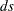data set.

Thedata set must have a _TYPE_ variable and contain the following two observations: a _TYPE_= 'LOCATION' observation and a _TYPE_= 'SCALE' observation. The variable _TYPE_ can also contain the optional observations, 'ADD' and 'MULT'; if these observations are not found in thedata set, the constants specified in the ADD= and MULT= options (or their default values) are used for unstandardization.

See the section OUTSTAT= Data Set for details about the statistics that each value of _TYPE_ represents. The formula used for unstandardization is as follows: If the final output value from the previous standardization is calculated as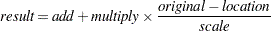The unstandardized variable is computed as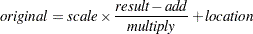VARDEF=DF | N | WDF | WEIGHT | WGT

specifies the divisor to be used in the calculation of variances. By default, VARDEF=DF. The values and associated divisors are as follows.

Value

Divisor

Formula

DF

Degrees of freedom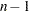N

Number of observations

n

WDF

Sum of weights minus 1

(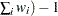WEIGHT | WGT

Sum of weights